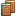##FREQUENTLY ASKED QUESTIONS

How is my rating calculated for multiplayer games?
The ratings system is based on statistical analysis using the Elo formula with a fixed K=10. Here is the formula we use:
 R'A = RA + 10(S-E) where RA is your current rating, S is the score (0=loss or 1=win) and E = 1 1 + 10(RMB - RMA)/400 RMA = RA - 100(M-1)S RMB = RB - 100(M-1)(1-S) where M is the multiplier (doubling cube value multiplied by 1=regular game, 2=gammon, or 3=backgammon), and RB is your opponent's current rating

Essentially, winning against a lower rated opponent yields a small rating increase but losing against a lower rated opponent yields a large rating decrease. Conversely, winning against a higher rated opponent yields a large rating increase but losing against a higher rated opponent yields a small rating decrease. In the case of the doubling cube, winning against a player with the same rating and the final cube value at 4 yields the same rating increase as winning a non-cube game against a player with a rating 400 points above yours.

All players start with a rating of 1500.

Games that end before each opponent makes 2 moves (short game) do not affect the winning player's rating and stats. The losing player's ratings and stats are always affected, regardless of the number of moves made.

See also FAQ #22 for more info on Gammons and Backgammons, and FAQ #31 for more on the Doubling Cube.
 [ List all questions and answers ]
©2008-2021  zooescape.com.   All rights reserved.
 backgammon • nackgammon • acey-deucey • sudoku • word games • chess